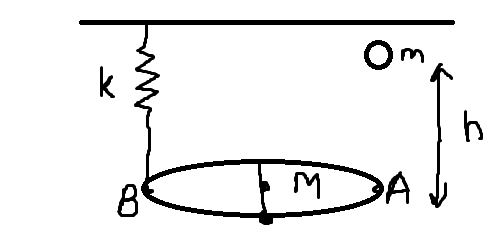# Need Help in MechanicsA horizontal disc of mass $M$ and radius $R$ is pivoted along one of its diameter and is free to rotate about it.A mass $m$ falls through height $h$ and sticks perfectly inelastically to the disc at point $A$. A spring of force constant $k$ is attached to point B of the disc as shown in figure. Assume $A,B$ are diametrically opposite points.Assume no heat dissipation ans the spring is initially in relaxed state.

I created this situation and I wish to examine it as practice. Can anyone please verify these equations?

Energy conservation: $mgh=\dfrac{1}{2}kx^2-mgx+\dfrac{1}{2}Iw^2$ (where $x$ is the distance through which the spring compresses and $w$ is angular velocity of disc)

Angular momentum conservation: $m\sqrt{2gh}R=Iw$ (since net torque zero initially)

$I=mR^2+\dfrac{MR^2}{4}$

Can somebody please improvise this and find more results? I think it may exhibit simple harmonic motion too (not able to prove)...Note by Ram Avasthi
3 years, 6 months ago

This discussion board is a place to discuss our Daily Challenges and the math and science related to those challenges. Explanations are more than just a solution — they should explain the steps and thinking strategies that you used to obtain the solution. Comments should further the discussion of math and science.

When posting on Brilliant:

• Use the emojis to react to an explanation, whether you're congratulating a job well done , or just really confused .
• Ask specific questions about the challenge or the steps in somebody's explanation. Well-posed questions can add a lot to the discussion, but posting "I don't understand!" doesn't help anyone.
• Try to contribute something new to the discussion, whether it is an extension, generalization or other idea related to the challenge.

MarkdownAppears as
*italics* or _italics_ italics
**bold** or __bold__ bold
- bulleted- list
• bulleted
• list
1. numbered2. list
1. numbered
2. list
Note: you must add a full line of space before and after lists for them to show up correctly
paragraph 1paragraph 2

paragraph 1

paragraph 2

[example link](https://brilliant.org)example link
> This is a quote
This is a quote
    # I indented these lines
# 4 spaces, and now they show
# up as a code block.

print "hello world"
# I indented these lines
# 4 spaces, and now they show
# up as a code block.

print "hello world"
MathAppears as
Remember to wrap math in $$ ... $$ or $ ... $ to ensure proper formatting.
2 \times 3 $2 \times 3$
2^{34} $2^{34}$
a_{i-1} $a_{i-1}$
\frac{2}{3} $\frac{2}{3}$
\sqrt{2} $\sqrt{2}$
\sum_{i=1}^3 $\sum_{i=1}^3$
\sin \theta $\sin \theta$
\boxed{123} $\boxed{123}$

Sort by:

Your main problem is that the spring does not remain vertical as the disc rotates. Suppose that the mass $m$ makes an angle $\theta$ with the horizontal. Then the length of the spring (assuming that its natural length is $L$) is $\sqrt{R^2(1-\cos\theta)^2 + (L-R\sin\theta)^2}$ and hence conservation of energy tells us that $\tfrac12k\big( \sqrt{L^2 - 2RL\sin\theta + 2R^2(1-\cos\theta)} - L\big)^2 - mgR\sin\theta + \tfrac12I\dot{\theta}^2$ is constant. For small oscillations, this means that $\tfrac12kR^2\theta^2 - mgR\theta + \tfrac12I\dot{\theta}^2$ is constant. Differentiating this gives you SHM in $\theta$.

You will get SHM, but only as an approximation for small values of $h$ (so that the oscillations are small).

- 3 years, 6 months ago

Oh right, I indeed forgot that spring won't remain vertical. Thanks for correcting. Also, what do you reckon with the angular momentum? Is it conserved?

- 3 years, 6 months ago

Conservation of angular momentum is fine, as you set it out, to describe the initial motion. As I said, though, either $h$ will be very small so that you only get small oscillations, or else you will need to solve the general differential equation in $\theta$ numerically - it is not likely that it will have a nice exact solution.

- 3 years, 6 months ago

- 3 years, 6 months ago